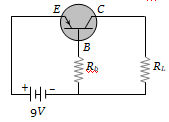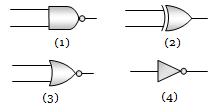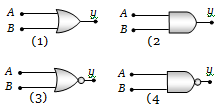In a transistor circuit shown here the base current is 35 $\mu$A. The value of the resistor Rb is(a) 123.5 k$\mathrm{\Omega }$
(b) 257 k$\mathrm{\Omega }$
(c) 380.05 k$\mathrm{\Omega }$
(d) None of these

Concept Questions :-

Transistor
High Yielding Test Series + Question Bank - NEET 2020

Difficulty Level:

For a transistor, in a common emitter arrangement, the alternating current gain $\mathrm{\beta }$ is given by

(a) $\mathrm{\beta }={\left(\frac{∆{\mathrm{I}}_{\mathrm{c}}}{∆{\mathrm{I}}_{\mathrm{B}}}\right)}_{{\mathrm{V}}_{\mathrm{C}}}$                       (b) $\mathrm{\beta }={\left(\frac{∆{\mathrm{I}}_{B}}{∆{\mathrm{I}}_{C}}\right)}_{{\mathrm{V}}_{\mathrm{C}}}$

(c) $\mathrm{\beta }={\left(\frac{∆{\mathrm{I}}_{\mathrm{c}}}{∆{\mathrm{I}}_{E}}\right)}_{{\mathrm{V}}_{\mathrm{C}}}$                       (d) $\mathrm{\beta }={\left(\frac{∆{\mathrm{I}}_{E}}{∆{\mathrm{I}}_{C}}\right)}_{{\mathrm{V}}_{\mathrm{C}}}$

Concept Questions :-

Transistor

Difficulty Level:

The relation between $\mathrm{\alpha }$ and $\mathrm{\beta }$ parameters of current gains for a transistors is given by

(a) $\mathrm{\alpha }=\frac{\mathrm{\beta }}{1-\mathrm{\beta }}$                (b) $\mathrm{\alpha }=\frac{\mathrm{\beta }}{1+\mathrm{\beta }}$

(c) $\mathrm{\alpha }=\frac{1-\mathrm{\beta }}{\mathrm{\beta }}$                (d) $\mathrm{\alpha }=\frac{1+\mathrm{\beta }}{\mathrm{\beta }}$

Concept Questions :-

Transistor
High Yielding Test Series + Question Bank - NEET 2020

Difficulty Level:

In the CB mode of a transistor, when the collector voltage is changed by 0.5 volt. The collector current changes by 0.05 mA. The output resistance will be
(a) 10 k$\mathrm{\Omega }$                         (b) 20 k$\mathrm{\Omega }$
(c) 5 k$\mathrm{\Omega }$                           (d) 2.5 k$\mathrm{\Omega }$

Concept Questions :-

Transistor
High Yielding Test Series + Question Bank - NEET 2020

Difficulty Level:

Consider an NPN transistor amplifier in the common-emitter configuration. The current gain of the transistor is 100. If the collector current changes by 1 mA, what will be the change in emitter current?
(a) 1.1 mA                     (b) 1.01 mA
(c) 0.01 mA                    (d) 10 mA

Concept Questions :-

Transistor
High Yielding Test Series + Question Bank - NEET 2020

Difficulty Level:

In a common base amplifier the phase difference between the input signal voltage and the output voltage is

(a) 0                      (b) $\mathrm{\pi }/4$

(c)$\mathrm{\pi }/2$                   (d) $\mathrm{\pi }$

Concept Questions :-

Transistor
High Yielding Test Series + Question Bank - NEET 2020

Difficulty Level:

In NPN transistor the collector current is 10 mA. If 90% of electrons emitted reach the collector, then

(a) Emitter current will be 9 mA
(b) Emitter current will be 11.1 mA
(c) Base current will be 0.1 mA
(d) Base current will be 0.01 mA

Concept Questions :-

Transistor

Difficulty Level:

In a transistor in CE configuration, the ratio of power gain to voltage gain is
(a) $\mathrm{\alpha }$                                      (b) $\mathrm{\beta }/\mathrm{\alpha }$
(c) $\mathrm{\beta \alpha }$                                     (d) $\mathrm{\beta }$

Concept Questions :-

Transistor
High Yielding Test Series + Question Bank - NEET 2020

Difficulty Level:

Given below are symbols for some logic gatesThe XOR gate and NOR gate respectively are
(a) 1 and 2                  (b) 2 and 3
(c) 3 and 4                  (d) 1 and 4

Concept Questions :-

Logic gates

Difficulty Level:

Given below are four logic gate symbol (figure). Those for OR, NOR and NAND are respectively(a) 1, 4, 3                     (b) 4, 1, 2
(c) 1, 3, 4                     (d) 4, 2, 1

Concept Questions :-

Logic gates